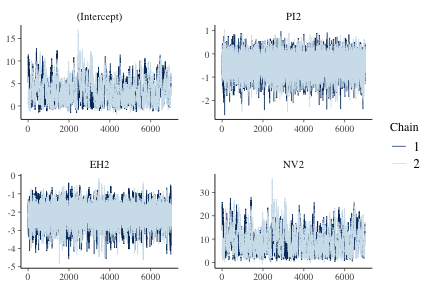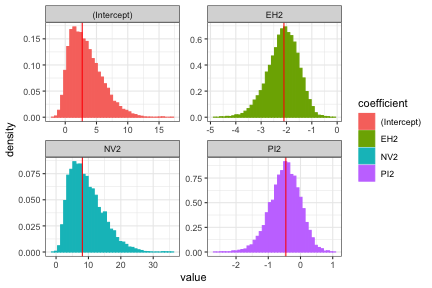# hmclearn: Logistic Regression Example

## Introduction

This vignette demonstrates fitting a logistic regression model via Hamiltonian Monte Carlo (HMC) using the hmclearn package.

library(hmclearn)
library(MCMCpack)

For binary response, we let

$p = Pr(\mathbf{y} = 1 | \mathbf{X}) = [1 + e^{-\mathbf{X}\boldsymbol\beta}]^{-1}.$

The vector of responses is $$\mathbf{y} = (y_1, ..., y_n)^T$$. The covariate values for subject $$i$$ are $$\mathbf{x}_i^T = (x_{i0}, ..., x_{iq})$$ for $$q$$ covariates plus an intercept. We write the full design matrix as $$\mathbf{X} = (\mathbf{x}_1^T, ..., \mathbf{x}_n^T)^T \in \mathbb{R}^{n\times(q+1)}$$ for $$n$$ observations. The regression coefficients are a vector of length $$q + 1$$, $$\boldsymbol\beta = (\beta_0, ..., \beta_q)^T$$.

## Derive log posterior and gradient for HMC

We develop the likelihood and log likelihood,

\begin{aligned} f(\mathbf{y} | \mathbf{X},\boldsymbol\beta) &= \prod_{i=1}^n p^{y_i} (1-p)^{1-y_i}, \\ &= \prod_{i=1}^{n} \left(\frac{1}{1+e^{-\mathbf{x}_i^T\boldsymbol\beta}}\right)^{y_i} \left(\frac{e^{-\mathbf{x}_i^T\boldsymbol\beta}}{1+e^{-\mathbf{x}_i^T\boldsymbol\beta}}\right)^{1-y_i}, \\ \log f(\mathbf{y} | \mathbf{X}, \boldsymbol\beta) &= \sum_{i=1}^n -y_i\log(1+e^{-\mathbf{x}_i^T\boldsymbol\beta}) + (1-y_i)(-\mathbf{x}_i\boldsymbol\beta - \log(1+e^{-\mathbf{x}_i^T\boldsymbol\beta})), \\ &= \sum_{i=1}^n -\log(1+e^{-\mathbf{x}_i\boldsymbol\beta}) - \mathbf{x}_i^T\boldsymbol\beta(1 - y_i), \\ &= \sum_{i=1}^n \mathbf{x}_i^T\boldsymbol\beta(y_i - 1) - \log(1 + e^{-\mathbf{x}_i^T\boldsymbol\beta}), \\ &= \boldsymbol\beta^T\mathbf{X}^T(\mathbf{y} - \mathbf{1}_n) - \mathbf{1}_n^T [ \log( 1 + e^{-\mathbf{X}\boldsymbol\beta})]. \end{aligned}

We set a multivariate normal prior for $$\boldsymbol\beta$$

\begin{aligned} \boldsymbol\beta &\sim N(0, \sigma_\beta^2 \mathbf{I}), \end{aligned}

with pdf, omitting constants

\begin{aligned} \pi(\boldsymbol\beta | \sigma_\beta^2) &= \frac{1}{\sqrt{\lvert 2\pi \sigma_\beta^2 \rvert }}e^{-\frac{1}{2}\boldsymbol\beta^T \boldsymbol\beta / \sigma_\beta^2}, \\ \log \pi(\boldsymbol\beta | \sigma_\beta^2) &= -\frac{1}{2}\log(2\pi \sigma_\beta^2) - \frac{1}{2}\boldsymbol\beta^T \boldsymbol\beta / \sigma_\beta^2, \\ &\propto -\frac{1}{2}\log \sigma_\beta^2 - \frac{\boldsymbol\beta^T\boldsymbol\beta}{2\sigma_\beta^2}. \end{aligned}

Next, we derive the log posterior, omitting constants,

\begin{aligned} f(\boldsymbol\beta | \mathbf{X}, \mathbf{y}, \sigma_\beta^2) &\propto f(\mathbf{y} | \mathbf{X}, \boldsymbol\beta) \pi(\boldsymbol\beta | \sigma_\beta^2), \\ \log f(\boldsymbol\beta | \mathbf{X}, \mathbf{y}, \sigma_\beta^2) & \propto \log f(\mathbf{y} | \mathbf{X}, \boldsymbol\beta) + \log \pi(\boldsymbol\beta|\sigma_\beta^2), \\ &\propto \sum_{i=1}^n \mathbf{x}_i^T\boldsymbol\beta(y_i - 1) - \log(1 + e^{-\mathbf{x}_i^T\boldsymbol\beta}) - \frac{1}{2}\boldsymbol\beta^T\boldsymbol\beta / \sigma_\beta^2, \\ &\propto \boldsymbol\beta^T\mathbf{X}^T(\mathbf{y} - \mathbf{1}_n) - \mathbf{1}_n^T[\log( 1 + e^{-\mathbf{X}\boldsymbol\beta})] - \frac{\boldsymbol\beta^T\boldsymbol\beta}{2\sigma_\beta^2}. \end{aligned}

Next, we need to derive the gradient of the log posterior for the leapfrog function

\begin{aligned} \nabla_{\boldsymbol\beta} \log f(\boldsymbol\beta, \mathbf{X}, \mathbf{y}, \sigma_\beta^2) &\propto \mathbf{X}^T \left ( \mathbf{y} - \mathbf{1}_n+ \frac{e^{-\mathbf{X}\boldsymbol\beta}}{1 + e^{-\mathbf{X}\boldsymbol\beta}}\right) - \boldsymbol\beta / \sigma_\beta^2 \end{aligned}

## Logistic Regression Example Data

The user must define provide the design matrix directly for use in hmclearn. Our first step is to load the data and store the design matrix $$X$$ and dependent variable vector $$y$$.

First, we load the Endometrial cancer data set (Heinze and Schember 2002) and create $$X$$ and $$y$$. This example also appears in Agresti (2015), and we compare results to his.

data(Endometrial)

# data prep
Endometrial$PI2 <- with(Endometrial, (PI - mean(PI)) / sd(PI)) Endometrial$EH2 <- with(Endometrial, (EH - mean(EH)) / sd(EH))
Endometrial$NV2 <- Endometrial$NV - 0.5

X <- cbind(1, as.matrix(Endometrial[, which(colnames(Endometrial)
%in% c("PI2", "EH2", "NV2"))]))
y <- Endometrial$HG colnames(X) <- c("(Intercept)", "PI2", "EH2", "NV2") ## Comparison models - Frequentist and Metropolis-Hastings To compare results, we first fit a standard linear model using the frequentist function glm. Note the high standard error estimates for the Intercept and NV2. This occurs because all 13 subjects with neovasculation present also have $$y_i = 1$$. See section 11.1.8 of Agresti (2015). f <- glm(y ~ X-1, family = binomial()) summary(f) #> #> Call: #> glm(formula = y ~ X - 1, family = binomial()) #> #> Deviance Residuals: #> Min 1Q Median 3Q Max #> -1.50137 -0.64108 -0.29432 0.00016 2.72777 #> #> Coefficients: #> Estimate Std. Error z value Pr(>|z|) #> X(Intercept) 7.8411 857.8755 0.009 0.992707 #> XPI2 -0.4217 0.4432 -0.952 0.341333 #> XEH2 -1.9219 0.5599 -3.433 0.000597 *** #> XNV2 18.1856 1715.7509 0.011 0.991543 #> --- #> Signif. codes: 0 '***' 0.001 '**' 0.01 '*' 0.05 '.' 0.1 ' ' 1 #> #> (Dispersion parameter for binomial family taken to be 1) #> #> Null deviance: 109.517 on 79 degrees of freedom #> Residual deviance: 55.393 on 75 degrees of freedom #> AIC: 63.393 #> #> Number of Fisher Scoring iterations: 17 As a result of challenges with the frequentist fit, Agresti (2015) in section 10.3.2 also fits this logistic regression model using a random walk Metropolis algorithm from the package (Martin, Quinn, Park 2011). We fit the model with the same number of MCMC iterations, prior distributions, and hyperparameters as in the text. This model also assigns a normal prior for $$\boldsymbol\beta$$ where $$\sigma_\beta^2 = 100$$. uses a precision parameterization, where $$B0 = 1/\sigma_\beta^2$$. The mean hyperparameter is 0 as specified in $$b0$$. Note the high number of Metropolis iterations specified in and the time required to fit, even with optimized compiled code. Our abbreviation MH refers to the general Metropolis-Hastings algorithm. set.seed(921) t1.mh <- Sys.time() fitMH <- MCMCpack::MCMClogit(HG ~ NV2 + PI2 + EH2, data=Endometrial, mcmc=10000000, b0=0, B0=0.01) t2.mh <- Sys.time() The time to fit the logistic regression using MH is t2.mh - t1.mh #> Time difference of 2.589012 mins We store the Metropolis parameter estimates in . (summMH <- summary(fitMH)) #> #> Iterations = 1001:10001000 #> Thinning interval = 1 #> Number of chains = 1 #> Sample size per chain = 1e+07 #> #> 1. Empirical mean and standard deviation for each variable, #> plus standard error of the mean: #> #> Mean SD Naive SE Time-series SE #> (Intercept) 3.2114 2.5585 0.0008091 0.0026811 #> NV2 9.1134 5.0921 0.0016103 0.0053685 #> PI2 -0.4723 0.4540 0.0001436 0.0006411 #> EH2 -2.1381 0.5935 0.0001877 0.0008742 #> #> 2. Quantiles for each variable: #> #> 2.5% 25% 50% 75% 97.5% #> (Intercept) -0.3453 1.2675 2.7207 4.6828 9.3351 #> NV2 2.1065 5.2285 8.1258 12.0409 21.3190 #> PI2 -1.4132 -0.7664 -0.4545 -0.1584 0.3664 #> EH2 -3.4015 -2.5152 -2.1004 -1.7222 -1.0815 ## Fit model using hmc Next, we fit the logistic regression model using HMC with the same hyperparameter for $$\sigma_\beta^2$$ as in Agresti’s example. N <- 1e4 set.seed(412) t1.hmc <- Sys.time() f_hmc <- hmc(N = N, theta.init = rep(1, 4), epsilon = 0.1, L = 20, logPOSTERIOR = logistic_posterior, glogPOSTERIOR = g_logistic_posterior, varnames = colnames(X), param=list(y = y, X=X, sig2beta=100), parallel=FALSE, chains=2) t2.hmc <- Sys.time() HMC requires fewer iterations and lower computation time than MH in this example. t2.hmc - t1.hmc #> Time difference of 39.86357 secs ## MCMC summary and diagnostics The acceptance ratio for each of the HMC chains is sufficiently high for an efficient simulation. f_hmc$accept/N
#>  0.9104 0.9155

The posterior quantiles are summarized after removing an initial burnin period. The $$\hat{R}$$ statistics provide an indication of convergence. Values close to one indicate that the multiple MCMC chains converged to the same distribution, while values above 1.1 indicate possible convergence problems. All $$\hat{R}$$ values in our example are close to one.

summary(f_hmc, burnin=3000, probs=c(0.025, 0.5, 0.975))
#> Summary of MCMC simulation
#>                  2.5%        50%      97.5%      rhat
#> (Intercept) -0.292832  2.9281285  9.2283365 1.0017419
#> PI2         -1.444876 -0.4606057  0.3592366 1.0000986
#> EH2         -3.423364 -2.1016785 -1.0784341 0.9999496
#> NV2          2.192343  8.5423329 21.0647490 1.0016373

Trace plots provide a visual indication of stationarity. These plots indicate that the MCMC chains are reasonably stationary.

mcmc_trace(f_hmc, burnin=3000)Histograms of the posterior distribution show that HMC parameter estimates align with Metropolis estimates from MCMCpack.

beta.mh <- summMH$quantiles[, "50%"][colnames(X)] diagplots(f_hmc, burnin=3000, comparison.theta=beta.mh) #>$histogram## Source

Heinze, G., & Schemper, M. (2002). A solution to the problem of separation in logistic regression. Statistics in medicine, 21(16), 2409-2419.

## Reference

Agresti, A. (2015). Foundations of linear and generalized linear models. John Wiley & Sons. ISBN: 978-1-118-73003-4

Martin AD, Quinn KM, Park JH (2011). “MCMCpack: Markov Chain Monte Carlo in R.” Journal of Statistical Software, 42(9), 22. https://www.jstatsoft.org/v42/i09/.

Thomas, Samuel, and Wanzhu Tu. “Learning Hamiltonian Monte Carlo in R.” arXiv preprint arXiv:2006.16194 (2020).